Courses

# Indifference Curve Analysis CA Foundation Notes | EduRev

## Business Economics for CA Foundation

Created by: Sushil Kumar

## CA Foundation : Indifference Curve Analysis CA Foundation Notes | EduRev

The document Indifference Curve Analysis CA Foundation Notes | EduRev is a part of the CA Foundation Course Business Economics for CA Foundation.
All you need of CA Foundation at this link: CA Foundation

INDIFFERENCE CURVE ANALYSIS
In the last section, we have discussed the marginal utility analysis of demand. A very popular alternative and a more realistic method of explaining consumer’s demand is the ordinal utility approach used a different tool namely indifference curve to analyse consumer behaviour. This approach to consumer behaviour is based on consumer preferences. It believes that human satisfaction, being a psychological phenomenon, cannot be measured quantitatively in monetary terms as was attempted in Marshall’s utility analysis. In this approach, it is felt that it is much easier and scientifically more sound to order preferences than to measure them in terms of money.
The consumer preference approach is, therefore, an ordinal concept based on ordering of preferences compared with Marshall’s approach of cardinality.
Assumptions Underlying Indifference Curve Approach
(i) The consumer is rational and possesses full information about all the relevant aspects of economic environment in which he lives.
(ii) The indifference curve analysis assumes that utility is only ordinally expressible. The consumer is capable of ranking all conceivable combinations of goods according to the satisfaction they yield. Thus, if he is given various combinations say A, B, C, D and E, he can rank them as first preference, second preference and so on. However, if a consumer happens to prefer A to B, he cannot tell quantitatively how much he prefers A to B.
(iii) Consumer’s choices are assumed to be transitive. If the consumer prefers combination A to B, and B to C, then he must prefer combination A to C. In other words, he has a consistent consumption pattern.
(iv) If combination A has more commodities than combination B, then A must be preferred to B.

Indifference Curves
What are Indifference Curves? The ordinal analysis of demand (here we will discuss the one given by Hicks and Allen) is based on indifference curves. An indifference curve is a curve which represents all those combinations of two goods which give same satisfaction to the consumer. Since all the combinations on an indifference curve give equal satisfaction to the consumer, the consumer is indifferent among them. In other words, since all the combinations provide the same level of satisfaction the consumer prefers them equally and does not mind which combination he gets.
If a consumer equally prefers two product bundles, then the consumer is indifferent between the two bundles. An Indifference curve is also called iso- utility curve or equal utility curve.
To understand indifference curves, let us consider the example of a consumer who has one unit of food and 12 units of clothing. Now, we ask the consumer how many units of clothing he is prepared to give up to get an additional unit of food, so that his level of satisfaction does not change. Suppose the consumer says that he is ready to give up 6 units of clothing to get an additional unit of food. We will have then two combinations of food and clothing giving equal satisfaction to the consumer: Combination A which has 1 unit of food and 12 units of clothing, and combination B which has 2 units of food and 6 units of clothing. Similarly, by asking the consumer further how much of clothing he will be prepared to forgo for successive increments in his stock of food so that his level of satisfaction remains unaltered, we get various combinations as given below:
Table 8 : Indifference Schedule

 Combination Food Clothing MRS A 1 12 B 2 6 6 C 3 4 2 D 4 3 1

Now, if we plot the above schedule, we will get the following figure.
In Figure 13, an indifference curve IC is drawn by plotting the various combinations given in the indifference schedule. The quantity of food is measured on the X axis and the quantity of clothing on the Y axis. As in indifference schedule, the combinations lying on an indifference curve will give the consumer the same level of satisfaction.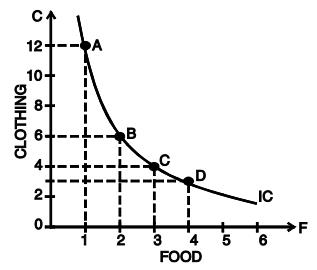Fig. 13 : A Consumer’s Indifference Curve

Indifference Map
An Indifference map represents a collection of many indifference curves where each curve represents a certain level of satisfaction. In short, a set of indifference curves is called an indifference map. An indifference map depicts the complete picture of consumer’s tastes and preferences. In Figure 14, an indifference map of a consumer is shown which consists of three indifference curves. We have taken good X on X-axis and good Y on Y-axis. It should be noted that while the consumer is indifferent among the combinations lying on the same indifference curve, he certainly prefers the combinations on the higher indifference curve to the combinations lying on a lower indifference curve because a higher indifference curve signifies a higher level of satisfaction. Thus, while all combinations of IC1 give him the same satisfaction, all combinations lying on IC2 give him greater satisfaction than those lying on IC1 .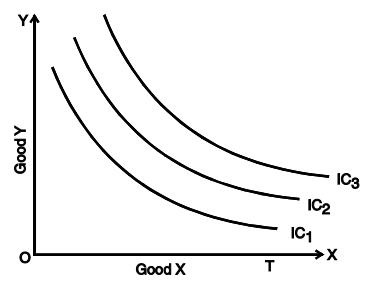Fig. 14 : Indifference Map

Marginal Rate of Substitution
Marginal Rate of Substitution (MRS) is the rate at which a consumer is prepared to exchange goods X and Y. Consider Table-8. In the beginning the consumer is consuming 1 unit of food and 12 units of clothing. Subsequently, he gives up 6 units of clothing to get an extra unit of food, his level of satisfaction remaining the same. The MRS here is 6. Likewise when he moves from B to C and from C to D in his indifference schedule, the MRS are 2 and 1 respectively. Thus, we can define MRS of X for Y as the amount of Y whose loss can just be compensated by a unit gain of X in such a manner that the level of satisfaction remains the same.
The marginal rate of substitution of X for Y (MRSxy) is equal to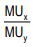We notice that MRS is falling i.e., as the consumer has more and more units of food, he is prepared to give up less and less units of clothing. There are two reasons for this.
1. The want for a particular good is satiable so that when a consumer has more of it, his intensity of want for it decreases. Thus, in our example, when the consumer has more units of food, his intensity of desire for additional units of food decreases.
2. Most goods are imperfect substitutes of one another. MRS would remain constant if they could substitute one another perfectly.

Properties of Indifference Curves
The following are the main characteristics or properties of indifference curves:
(i) Indifference curves slope downward to the right: This property implies that the two commodities can be substituted for each other and when the amount of one good in the combination is increased, the amount of the other good is reduced. This is essential if the level of satisfaction is to remain the same on an indifference curve.
(ii) Indifference curves are always convex to the origin : It has been observed that as more and more of one commodity (X) is substituted for another (Y), the consumer is willing to part with less and less of the commodity being substituted (i.e. Y). This is called diminishing marginal rate of substitution. Thus, in our example of food and clothing, as a consumer has more and more units of food, he is prepared to forego less and less units of clothing. This happens mainly because the want for a particular good is satiable and as a person has more and more of a good, his intensity of want for that good goes on diminishing. In other words, the subjective value attached to the additional quantity of a commodity decreases fast in relation to the other commodity whose total quantity is decreasing. This diminishing marginal rate of substitution gives convex shape to the indifference curves. However, there are two extreme situations. When two goods are perfect substitutes of each other, the indifference curve is a straight line on which MRS is constant. And when two goods are perfect complementary goods (e.g. printer and cartridge), the indifference curve will consist of two straight lines with a right angle bent which is convex to the origin, or in other words, it will be L shaped
(iii) Indifference curves can never intersect each other:No two indifference curves will intersect each other although it is not necessary that they are parallel to each other. In case of intersection the relationship becomes logically absurd because it would show that higher and lower levels are equal, which is not possible. This property will be clear from Figure 15.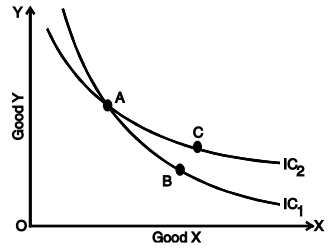Fig. 15 : Intersecting Indifference Curves

In gure 15, IC1 and IC2 intersect at A. Since A and B lie on IC1 , they give same satisfaction to the consumer. Similarly since A and C lie on IC2 , they give same satisfaction to the consumer. This implies that combination B and C are equal in terms of satisfaction. But a glance will show that this is an absurd conclusion because certainly combination C is better than combination B because it contains more units of commodities X and Y. Thus we see that no two indifference curves can touch or cut each other.
(iv) A higher indifference curve represents a higher level of satisfaction than the lower indifference curve: This is because combinations lying on a higher indifference curve contain more of either one or both goods and more goods are preferred to less of them.
(v) Indifference curve will not touch either axes: Another characteristic feature of indifference curve is that it will not touch the X axis or Y axis. This is born out of our assumption that the consumer is considering different combination of two commodities. If an indifference curve touches the Y axis at a point P as shown in the figure 16, it means that the consumer is satisfied with OP units of y commodity and zero units of x commodity. This is contrary to our assumption that the consumer wants both commodities although in smaller or larger quantities. Therefore an indifference curve will not touch either the X axis or Y axis.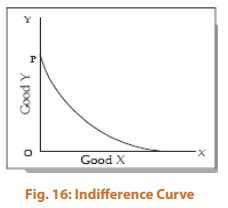,

,

,

,

,

,

,

,

,

,

,

,

,

,

,

,

,

,

,

,

,

;